• (j, i) //表示的是 j 行 i 列 的这个像素 srcImage.at<uchar>(Point(j, i)) //表示的是 坐标（j,i）的像素 1 2 为了我下面的图方便说明 j 换成 x , i 换成 y 。也就是说： srcImage.at<uchar>(x, y) //...
我说说我的理解：
srcImage.at<uchar>(j, i) //表示的是  j 行 i 列 的这个像素 srcImage.at<uchar>(Point(j, i)) //表示的是 坐标（j,i）的像素 1 2 为了我下面的图方便说明 j 换成 x , i 换成 y 。也就是说：
srcImage.at<uchar>(x, y) //表示的是 x 行 y 列 的这个像素 srcImage.at<uchar>(Point(x, y)) //表示的是 坐标（x,y）的像素 1 2 可能就会有人不明白了，有什么区别呢？？  先上一幅图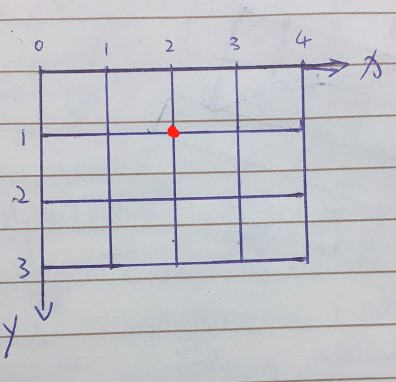问图中红点的坐标是？ （2，1） 还是 （1，2）？  答案是（2，1）！  为什么？ 看清了 我问的是坐标！！  那么（2，1） 和 （1，2）究竟有什么不同？  （2，1）表示的是红点的坐标，就是红点的 x 坐标是 2， y 坐标是 1。  （1，2）表示的是红点的行和列，就是红点的行是1，列是2，也就是红点在第 1 行，第 2 列。  这下子我相信大家都明白这两者的区别了吧
srcImage.at<uchar>(j, i) srcImage.at<uchar>(Point(j, i))
简单而言：.at()是将图像上的像素作为矩阵去操作，（x,y）表示的是x行y列
而Point（x,y）是按照图像的坐标系，原点在左上角。
展开全文• import numpy as np import cv2 as cv import matplotlib.pyplot as plt img = cv.imread(‘D:/lene.jpg’,1) px = img[400,400] print(px) ROI = img[280:340,330:390] #选取ROI img[273:333,100:160] = ROI #图像...
import numpy as np import cv2 as cv import matplotlib.pyplot as plt
img = cv.imread(‘D:/lene.jpg’,1) px = img[400,400] print(px) ROI = img[280:340,330:390] #选取ROI img[273:333,100:160] = ROI #图像的原始区域用ROI代替
b,g,r = cv.split(img) #分裂 img2 = cv.merge((r,g,b)) #合并
blue = img[:,:,0] #代表B通道，也就是蓝色分量图像 green = img[:,:,1]#代表G通道，也就是绿色分量图像； red = img[:,:,2] #代表R通道，也就是红色分量图像；
img3 = cv.merge((red,green,blue)) #合并
cv.imshow(‘image’,img) plt.subplot(121);plt.imshow(img2) plt.subplot(122);plt.imshow(img3) plt.show()
k = cv.waitKey(0) if k == 27: cv.destroyAllWindows() elif k == ord(‘s’): cv.imwrite(‘lena.jpg’,img) cv.destroyAllWindows() 显示结果：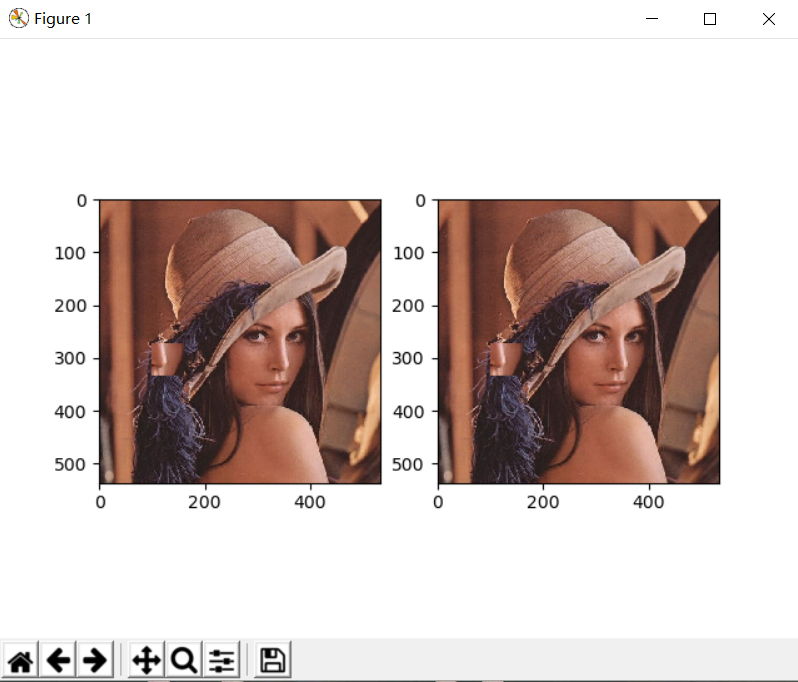展开全文计算机视觉
• 距离变换的基本意思就是计算一个图像中非零像素点到最近的零像素点的距离，也就是到所有零像素点的最短距离。建立距离变换图像可以方便提取骨骼 在opencv中有专门的函数cvDistTransform来计算距离变换图像。 ...
当时自己理解的一点小误区，放到这里防止再犯错。
距离变换的基本意思就是计算一个图像中非零像素点到最近的零像素点的距离，也就是到所有零像素点的最短距离。建立距离变换图像可以方便提取骨骼
在opencv中有专门的函数cvDistTransform来计算距离变换图像。

DistTransform
计算输入图像的所有非零元素对其最近零元素的距离

void cvDistTransform( const CvArr* src, CvArr* dst, int distance_type=CV_DIST_L2,
src
输入 8-比特、单通道 (二值) 图像.
dst
含计算出的距离的输出图像(32-比特、浮点数、单通道).
distance_type
距离类型; 可以是 CV_DIST_L1, CV_DIST_L2, CV_DIST_C 或 CV_DIST_USER.
距离变换掩模的大小，可以是 3 或 5. 对 CV_DIST_L1 或 CV_DIST_C 的情况，参数值被强制设定为 3, 因为 3×3 mask 给出 5×5 mask 一样的结果，而且速度还更快。
函数 cvDistTransform 二值图像每一个象素点到它最邻近零象素点的距离。对零象素，函数设置 0 距离，对其它象素，它寻找由基本位移（水平、垂直、对角线或knight's move，最后一项对 5×5 mask 有用）构成的最短路径。 全部的距离被认为是基本距离的和。由于距离函数是对称的，所有水平和垂直位移具有同样的代价 (表示为 a ), 所有的对角位移具有同样的代价 (表示为 b), 所有的 knight's 移动具有同样的代价 (表示为 c). 对类型 CV_DIST_C 和 CV_DIST_L1，距离的计算是精确的，而类型 CV_DIST_L2 (欧式距离) 距离的计算有某些相对误差 (5×5 mask 给出更精确的结果), OpenCV 使用 [Borgefors86] 推荐的值：

CV_DIST_C (3×3):
a=1, b=1

CV_DIST_L1 (3×3):
a=1, b=2

CV_DIST_L2 (3×3):
a=0.955, b=1.3693

CV_DIST_L2 (5×5):
a=1, b=1.4, c=2.1969

距离计算
2b?2a b?bab?2aa0a2a?bab?b?a?b
a\b分别表示在水平垂直方向的距离，？/问号部分表示根据a和b的值的大小再确定其值。

a,b,c
2bc2ac2bcbabc2aa0a2acbabc2bc2ac2b

下面代码表示自己创建一个9*9的矩阵，然后利用该函数计算其值：

//距离变换代码，在微软的那个ppt中用来计算掌心的位置可能用到这个
//部分内容在http://www.opencv.org.cn/forum/viewtopic.php?f=1&t=4574&start=0有解释

#include <iostream>
#include "highgui.h"
#include "cv.h"

using namespace std;

int main()
{

IplImage *src = cvCreateImage(cvSize(9, 9), 8, 1);
cvZero(src);
uchar *ptr = (uchar*)(src->imageData);
*ptr++ = 0;*ptr++ = 0;*ptr++ = 0;*ptr++ = 0;*ptr++ = 0;*ptr++ = 0;*ptr++ = 0; *ptr++ = 0;*ptr++ = 0;ptr +=3;
*ptr++ = 0;*ptr++ = 255;*ptr++ = 255;*ptr++ = 255;*ptr++ = 255;*ptr++ = 0;*ptr++ = 0; *ptr++ = 0;*ptr++ = 0;ptr +=3;
*ptr++ = 0;*ptr++ = 255;*ptr++ = 255;*ptr++ = 255;*ptr++ = 255;*ptr++ = 255;*ptr++ = 255; *ptr++ = 0;*ptr++ = 0;ptr +=3;
*ptr++ = 0;*ptr++ = 0;*ptr++ = 255;*ptr++ = 255;*ptr++ = 255;*ptr++ = 255;*ptr++ = 255;*ptr++ = 0;*ptr++ = 0;ptr +=3;
*ptr++ = 0;*ptr++ = 255;*ptr++ = 255;*ptr++ = 255;*ptr++ = 255;*ptr++ = 255;*ptr++ = 255;*ptr++ = 255;*ptr++ = 0;ptr +=3;
*ptr++ = 0;*ptr++ = 0;*ptr++ = 255;*ptr++ = 255;*ptr++ = 255;*ptr++ = 255;*ptr++ = 255;*ptr++ = 0;*ptr++ = 0;ptr +=3;
*ptr++ = 0;*ptr++ = 0;*ptr++ = 255;*ptr++ = 255;*ptr++ = 255;*ptr++ = 255;*ptr++ = 255; *ptr++ = 0;*ptr++ = 0;ptr +=3;
*ptr++ = 0;*ptr++ = 0;*ptr++ = 255;*ptr++ = 0;*ptr++ = 255;*ptr++ = 0;*ptr++ = 0;*ptr++ = 0;*ptr++ = 0; ptr +=3;
*ptr++ = 0;*ptr++ = 0;*ptr++ = 0;*ptr++ = 0;*ptr++ = 0;*ptr++ = 0;*ptr++ = 0;*ptr++ = 0;*ptr++ = 0;

IplImage *dist = cvCreateImage( cvGetSize(src), IPL_DEPTH_32F, 1 );
cvDistTransform( src, dist, CV_DIST_L1, 3, NULL, NULL );
/*cvConvertScale( dist, dist, 1000.0, 0 );
cvShowImage("dist2", dist);
cvPow( dist, dist, 0.5 );
cvShowImage("dist3", dist);
IplImage *dist32s = cvCreateImage(cvGetSize(src), IPL_DEPTH_32S, 1);
cvConvertScale( dist, dist32s, 1.0, 0.5 );
cvShowImage("dist32s", dist32s);
cvAndS( dist32s, cvScalarAll(255), dist32s, 0 );
cvShowImage("dist32s2", dist32s);
IplImage *dist8u1 = cvCloneImage(src);
cvConvertScale( dist32s, dist8u1, 1, 0 );
cvShowImage("dist8u1", dist8u1);*/

for (int y=0; y<src->height; y++)
{
uchar *pt = (uchar*)(src->imageData+y*src->widthStep);
for (int x=0; x<src->width; x++)
{
cout << int(pt[x]) << '\t';
}
cout << endl;
}
cout << endl << endl;
for (int y=0; y<dist->height; y++)
{
float *p = (float *)(dist->imageData+y*dist->widthStep);//因为输出图像必须是浮点型数据，所以这里必须采用float。也是自己一开始做错的。虽然IplImage->imageData是char型的，但是针对不同类型（字节型浮点型）要有不同的处理方式
for (int x=0; x<dist->width; x++)
{
cout << float(p[x]) << '\t';
}
cout << endl;
}

cvShowImage("src",src);
cvShowImage("dist", dist);
cvWaitKey(0);
return 0;

}运行结果如果将距离运算改为CV_DIST_L2运行结果：因为一开始栽倒数据访问上了，所以有必要将opencv中像素访问方式贴出来，方便以后查阅。csnd编辑界面太差了，一堆乱码，大家还是移步到:http://www.opencv.org.cn/index.php/OpenCV_编程简介（矩阵/图像/视频的基本读写操作）
展开全文distance float null c dst
• Opencv像素处理与访问 对于图像处理来说，如果我们了解处理图像的具体算法，那么我们就可以通过直接操作图片的像素点来实现这些算法。所以本文就总结一下Opencv像素处理和访问的一些知识。 文章目录Opencv像素处理...
• OpenCV像素点邻域遍历效率比较，以及访问像素点的几种方法
OpenCV像素点邻域遍历效率比较，以及访问像素点的几种方法
前言：
以前笔者在项目中经常使用到OpenCV的算法，而大部分OpenCV的算法都需要进行遍历操作，而且很多遍历操作都是需要对目标像素点的邻域进行二次遍历操作。笔者参考了很多博文，经过了实验，在这篇博文中总结了OpenCV的遍历操作的效率。
参考博文：  《OpenCV获取与设置像素点的值的几个方法 》  《【OpenCV】访问Mat中每个像素的值（新）》  《[OpenCV] 访问Mat矩阵中的元素》  《OpenCV学习笔记（四十二）——Mat数据操作之普通青年、文艺青年、暴力青年》  《OpenCV学习笔记（四十三）——存取像素值操作汇总core》  《图像噪声的抑制——均值滤波、中值滤波、对称均值滤波》
一. 均值滤波
由于笔者想要了解像素点及其邻域的遍历，所以本文用于测试的算法是均值滤波。  均值滤波的方法比较简单。对待需要处理的当前像素，选择一个模板，该模板为其邻域内若干像素，然后用模板内所有像素的均值来替代原像素值。公式如下：均值滤波模板如下图所示：图中的模板大小选择了3 × 3矩阵，图中的1–8绿色部分的像素都是邻域内像素，黄色像素是在图像(x, y)像素处的均值滤波结果。
该方法十分简单，包括了简单的邻域像素点的操作，优缺点也十分明显：
优点：算法简单，计算速度快；缺点：降低噪声的同时使图像产生模糊，特别是景物的边缘和细节部分。
二. 邻域遍历方法
笔者对不同邻域遍历方法使用的算法与图片如下：
测试算法：均值滤波滤波内核尺寸：3 × 3图片尺寸：580 × 410
原图如下：笔者参考了博文《【OpenCV】访问Mat中每个像素的值（新）》，从其中学习了几种遍历像素点的方法。针对邻域遍历，笔者最后总结了三种方法如下：
1. ptr与[]
Mat最直接的访问方法，是通过.ptr<>函数得到一行的指针，并用[]操作符访问某一列的像素值。  源码如下：
// using .ptr and []
void MyBlur_1(Mat src, Mat& dst)
{
dst.create(src.size(), src.type());
// 强制将ksize变为奇数
//   int ksize = ksize / 2 * 2 + 1;
int ksize = 3;
// kernel的半径
//    kr = ksize / 2;
int kr = 1;

int nr = src.rows - kr;
int nc = (src.cols - kr) * src.channels();

for(int j = kr; j < nr; j++)
{
// 获取图像三行数据的地址
const uchar* previous = src.ptr<uchar>(j - 1);
const uchar* current = src.ptr<uchar>(j);
const uchar* next = src.ptr<uchar>(j + 1);

uchar* output = dst.ptr<uchar>(j);

for(int i = 1 * src.channels(); i < nc; i++)
{
output[i] = cv::saturate_cast<uchar>(
(previous[i - src.channels()] + previous[i] + previous[i + src.channels()]
+ current[i - src.channels()] + current[i] + current[i + src.channels()]
+ next[i - src.channels()] + next[i] + next[i + src.channels()]) / 9);
}
}
}
2. at获取图像坐标
.at操作可以用于操作单个像素点。通常的操作如下：
// 对于单通道图像
img.at<uchar>(i, j) = 255;
// 对于多通道图像
img.at<cv::Vec3b>(i, j) = 255;
用at实现均值滤波的代码如下：
// using at
void MyBlur_2(Mat src, Mat& dst)
{
dst.create(src.size(), src.type());
// 强制将ksize变为奇数
//   int ksize = ksize / 2 * 2 + 1;
int ksize = 3;
// kernel的半径
//    kr = ksize / 2;
int kr = 1;

int nr = src.rows - kr;
int nc = src.cols - kr;

for(int j = kr; j < nr; j++)
{
for(int i = src.channels(); i < nc; i++)
{
dst.at<Vec3b>(j, i) = cv::saturate_cast<uchar>(
(src.at<Vec3b>(j-1, i-1) + src.at<Vec3b>(j, i-1) + src.at<Vec3b>(j+1, i-1)
+ src.at<Vec3b>(j-1, i) + src.at<Vec3b>(j, i) + src.at<Vec3b>(j+1, i)
+ src.at<Vec3b>(j-1, i+1) + src.at<Vec3b>(j, i+1) + src.at<Vec3b>(j+1, i+1)) / 9 );
dst.at<Vec3b>(j, i) = cv::saturate_cast<uchar>(
(src.at<Vec3b>(j-1, i-1) + src.at<Vec3b>(j, i-1) + src.at<Vec3b>(j+1, i-1)
+ src.at<Vec3b>(j-1, i) + src.at<Vec3b>(j, i) + src.at<Vec3b>(j+1, i)
+ src.at<Vec3b>(j-1, i+1) + src.at<Vec3b>(j, i+1) + src.at<Vec3b>(j+1, i+1)) / 9 );
dst.at<Vec3b>(j, i) = cv::saturate_cast<uchar>(
(src.at<Vec3b>(j-1, i-1) + src.at<Vec3b>(j, i-1) + src.at<Vec3b>(j+1, i-1)
+ src.at<Vec3b>(j-1, i) + src.at<Vec3b>(j, i) + src.at<Vec3b>(j+1, i)
+ src.at<Vec3b>(j-1, i+1) + src.at<Vec3b>(j, i+1) + src.at<Vec3b>(j+1, i+1)) / 9 );
}
}
}
然而，Debug版本下，at操作要比指针的操作慢很多，所以对于不连续数据或者单个点处理，可以考虑at操作，对于连续的大量数据，尽量不要使用它。
Release版本与Debug版本的对比，以及at操作的效率，在后面会有比较。
3. data
Mat类中，对data的定义如下：
//! pointer to the data
uchar* data;
data是Mat对象中的指针，指向存放内存中存放矩阵数据的一块内存，类型为uchar*。  又由于二维矩阵Mat中任一像素的地址为：

addr(M_{i,j}) = M.data + M.step \times i + M.step \times j
计算得到的便是M矩阵中像素(i, j)的地址。而又有：

M.step=M.cols×M.channels
$M.step = M.cols \times M.channels$

M.step=M.channels
$M.step = M.channels$
所以上式可以改写为：

addr(M_{i,j}) = M.data + M.cols \times M.channels \times i + M.channels \times j

综上，用.data实现均值滤波的代码如下：
// using .data
void MyBlur_3(Mat src, Mat& dst)
{
dst.create(src.size(), src.type());
// 强制将ksize变为奇数
//   int ksize = ksize / 2 * 2 + 1;
int ksize = 3;
// kernel的半径
//    kr = ksize / 2;
int kr = 1;

int nr = src.rows - kr;
int nc = (src.cols - kr) * src.channels();
uchar* srcdata = (uchar*)src.data;
uchar* dstdata = (uchar*)dst.data;

for(int j = kr; j < nr; j++)
{
uchar* psrc_0 = srcdata + (j-1) * src.cols * src.channels();
uchar* psrc_1 = srcdata + j * src.cols * src.channels();
uchar* psrc_2 = srcdata + (j+1) * src.cols * src.channels();
uchar* pdst = dstdata + j * dst.cols * dst.channels();
for(int i = src.channels(); i < nc; i++)
{
*pdst++ = (psrc_0[i - src.channels()] + psrc_0[i] + psrc_0[i + src.channels()]
+ psrc_1[i - src.channels()] + psrc_1[i] + psrc_1[i + src.channels()]
+ psrc_2[i - src.channels()] + psrc_2[i] + psrc_2[i + src.channels()]) / 9;
}
}
}
需要注意的是，前面说的at操作与ptr操作，都是带有内存检查，防止操作越界的，然而使用data指针比较危险，虽然在Debug版本下的速度确实让人眼前一亮。所以在《Mat数据操作之普通青年、文艺青年、暴力青年》中，博主将.data操作称之为暴力青年。
三. 不同方法效率比较
写这篇博文之前，我还以为不同的方法对效率的影响十分巨大。当然这种想法是没有错的，因为在Debug版本中确实不同的遍历方法有着很大的效率区别。但是看了博客《Mat数据操作之普通青年、文艺青年、暴力青年》中的总结后，笔者才意识到原来在Release版本下，上述方法的效率其实差别不大。
1. 测试源码：
#include "cv.h"
#include "highgui.h"
#include <vector>
#include <cmath>
#include <math.h>
#include <iostream>

using namespace cv;
using namespace std;

void MyBlur_1(Mat src, Mat& dst);
void MyBlur_2(Mat src, Mat& dst);
void MyBlur_3(Mat src, Mat& dst);

// 测试遍历像素点对均值滤波的效率
int main()
{
double time;
double start;

Mat img;

Mat dst1;
start = static_cast<double>(getTickCount());
MyBlur_1(img, dst1);
time = ((double)getTickCount() - start) / getTickFrequency() * 1000;
cout << "using .ptr and []"<<endl;
cout << "Time: " << time << "ms" << endl<<endl;

Mat dst2;
start = static_cast<double>(getTickCount());
MyBlur_2(img, dst2);
time = ((double)getTickCount() - start) / getTickFrequency() * 1000;
cout << "using at "<<endl;
cout<< "Time: " << time << "ms" << endl <<endl;

Mat dst4;
start = static_cast<double>(getTickCount());
MyBlur_3(img, dst4);
time = ((double)getTickCount() - start) / getTickFrequency() * 1000;
cout <<"using .data"<<endl;
cout << "Time: " << time << "ms" << endl<<endl;

Mat dst3;
start = static_cast<double>(getTickCount());
blur(img, dst3, Size(3, 3));
time = ((double)getTickCount() - start) / getTickFrequency() * 1000;
cout<<"using OpenCV's blur"<<endl;
cout << "Time: " << time << "ms" <<endl << endl;

imshow("src", img);
imshow("using .ptr and []", dst1);
imshow("using at ", dst2);
imshow("using .data", dst4);
waitKey(0);

return 0;
}
2. Debug版本
运行结束后，结果输出如下：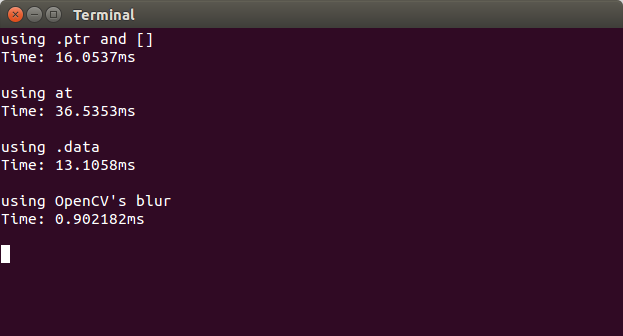如图所示，在Debug版本下，不同的遍历方法对遍历的效率影响还是很大的。值得注意的有下面几点：
(1) 检查操作对效率的影响
实际上，at操作符与ptr操作符在Debug版本下都是有内存检查、防止操作越界的操作，而data十分简单粗暴，没有任何检查，由于它的简单粗暴所以使得data操作速度很快。所以在Debug版本下，at操作符与ptr操作符相较于data，速度还是慢了不少。  另外在Debug版本下，at操作要比指针操作慢得多，所以对于不连续数据或者单个点处理，可以考虑at操作，对于连续的大量数据，尽量不要使用它。
(2) 对重复计算进行优化
在博文《OpenCV学习笔记（四十三）——存取像素值操作汇总core 》中，博主提到：“在循环中重复计算已经得到的值，是个费时的工作。”并做了对比：
    int nc = img.cols * img.channels();
for (int i=0; i<nc; i++)
{.......}

//**************************

for (int i=0; i<img.cols * img.channels(); i++)
{......}  
博主说经过他的测试，前者明显快于后者。  笔者发现这正好是笔者的编程风格，躺着中了一枪…… 那么如果笔者把这一点改了，是不是也会有比较好的效率提升呢？作死的笔者尝试了一下：  在没有更改for循环之前，Debug版本的效率是这样的：之后笔者对所有for循环做了上面的优化，测试结果如下：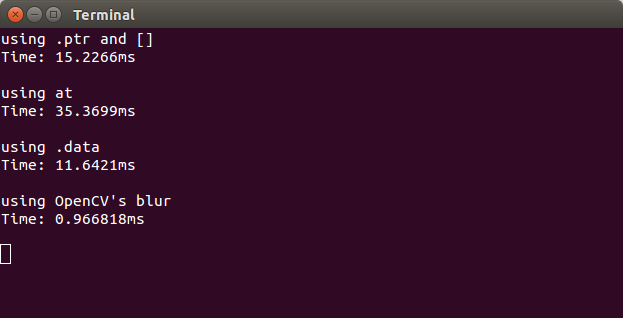结果显示，确实对于这几种遍历方式都是有一定的提升效果的。
3. Release版本
笔者尝试运行了一下Release版本，结果如下：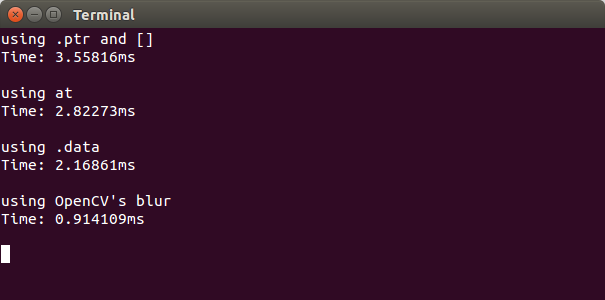作对比如下：
MethodDebug(ms)Release(ms)ptr15.2263.5582at35.3702.8227data11.6422.1686
的确，Release版本下的遍历方法基本上效率都差不多，尤其是at，本来速度应该最慢，但在Release版本下也有很快的速度。这是由于Mat::at()操作在Debug模式下加入了CV_DbgAssert()来判断一些条件，所以拖慢了函数的运行；而在Release版本下没有了CV_DbgAssert()，所以速度有了提升。
四. 其他遍历像素点的方法
笔者推荐博文《【OpenCV】访问Mat中每个像素的值（新） 》，博主在文中提出了十余种遍历像素点的方法，且在文章最后给出了各种方法的运行效率，可谓十分详细，所以笔者在此就不赘述了。  注：博主的对不同方法的比较，在评论区也被指出都是在Debug版本下的对比，如果将程序调整至Release版本，各个方法的效率也没有太大差别。
展开全文遍历 运行效率
• 1. 访问像素的三种方法 ①指针访问：最快 ②迭代器iterator：较慢，非常安全，指针访问可能出现越界问题 ③动态地址计算：更慢，通过at()实现。适用于访问具体某个第i行，j列的像素，而不适用遍历像素 2. Mat...
• 环境配置   VS2013+OpenCV3.0+Win7（X64）    前言 ...所以我们需要了解一下OpenCV如何访问图像中的像素点。首先说明一下，这里默认图像储存为Mat格式，RGB类型。    三种访问方式 ...
• 代码讲解请看本人博客关于opencv访问像素的五种方法。
• a.使用指针 #include <opencv.hpp> using namespace cv; using namespace std;... //指针访问每个像素并赋值 Mat img = imread("001.jpg"); for (int i = 0; i < img.rows; i++) ...
• 点击上方“小白学视觉”，选择加"星标"或“置顶”重磅干货，第一时间送达 本文转自：opencv学堂Python OpenCV像素操作环境声明：Python3.6 + ...
• 像素存储 OpenCV 中图像矩阵的大小取决于所用的颜色模型，更准确的说是取决于图像所用到的通道数。 如果使用的是灰度图，矩阵大概如图所示： 如果使用的是多通道的图像，矩阵中的列会包含多个子列，子列的个数和...
• 1.存取单个像素值 img.at(i,j) = 255; img.at(i,j) = 255;//这里有个不知道为什么，现在先这样记住 如果你觉得at操作显得太笨重了，不想用Mat这个类，也可以考虑使用轻量级的Mat_类，使用重载操作符()实现...iterator 优化 多线程 image 测试
• opencv中有3中方法可以访问/修改图像的像素值，分别为：1. 指针访问2. 迭代器iterator3. 动态地址计算 测试程序如下：[cpp] view plain copy#include "opencv2/opencv.hpp" #include "...
• 本人比较习惯于Opencv1的代码写法，看了最新一本关于Opencv2写法，虽然原理是一样的但是写法差别是很大的，所以我看了Opencv2访问像素写法后就尝试改变为Opencv1代码写法。 下面代码是网上的：修改图片的对比度与...
• opencv OpenCV访问图像像素, 数组元素等方法收集 分类： OPENCV2012-10-08 21:13 104人阅读 评论(0) 收藏 举报 imagefloatbyteaccesswrapperalignment [cpp] view plaincopy ...
• opencv中三种访问像素的方法要，使用at访问单通道的图像，注意其顺序 img.at(x,y)，访问的其实是图像中(y,x)点； img.at(Point(x,y)),访问的才是我们希望的（x,y）点像素； 原因的oencv Mat中存储的像素点下标...错误
• 首次接触图像处理，通过次来记录自己的学习记录，随着了解的越多，可能就会有不同的理解，到时再来补充。通过对毛星云大大例程的操作，指针、迭代、动态地址访问之间最大的区别就是，时间的消耗会越来越大
• 1.用Numpy库生成一个元素值都是0的二维数组，用来模拟一幅黑色图像，并对其进行访问、修改。 分析：使用Numpy库中的函数zeros()可以生成一个元素值都是0的数组，并可以直接使用数组的索引对其进行访问、修改。 ...
• 我想问的是mat的ptr函数只能按行访问数据元素吗 /* .ptr with [] */ for ( int i=0; i; i++ ) { uchar *data = image.ptr( i ); for ( int j=0; j; j++ ) { data[j] = 0; /* if color one: data[j*3 + 0] = 0...c++
• OpenCV2 访问图像的各个像素有各种方法 我们来用各种方法来实现减少图像的颜色数量 color = color/div*div +div/2; 若div为8，则原来RGB每个通道的256种颜色减少为32种。 若div为64，则原来RGB每
• opencv 中的像素访问主要有三种方式：下标访问，指针访问，迭代器访问。 //下标方式 void colorReduce(Mat& inputImage, Mat& outputImage, int div) { //参数准备 outputImage = inputImage.clone(); /...
• Opencv访问图像像素源码 实例图像 C++代码
• 这个操作跟上面的就有点区别了，需要对这个像素三个通道的每个对应元素赋值,Opencv中图像三原色在内存中的排列顺序为B-G-R（见下面注释），操作过程如下： img.at(14,25) = 25; img.at(14,25) = 25; img....
• 比较简单，不必过多赘述，用到了过来看看 #include "opencv2/opencv.hpp" #include <iostream>... ////动态地址访问 //Mat img = imread("E:\\OpenCV\\images\\fqy1.jpg"); //imshow("src", img); /
• saturate_cast用于防止数据溢出。 优质博客： ...utm_term=opencv%E5%83%8F%E7%B4%A0%E8%A1%A8%E7%A4%BA&a计算机视觉
• 出现如下图的断言错误，说明你的图片的类型和at时的类型不对 如：我访问的图像是CV_64F的，而我的访问写的是 src.at(row,col)发生错误。 解决办法： 将访问改写成src.at(row,col) Opencv中对应的类型如下： ......